# Advanced Select Statements Select List Variations SELECT Column

• Slides: 13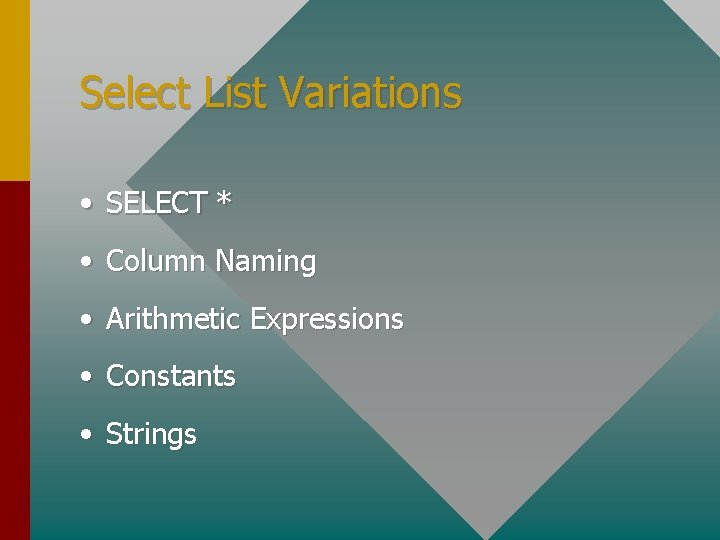Select List Variations • SELECT * • Column Naming • Arithmetic Expressions • Constants • StringsColumn Naming Using column aliases we can control how the column names are displayed in our output. SELECT au_fname First_Name FROM authors The output will reflect this column name. First_Name -------Joe Dan Sue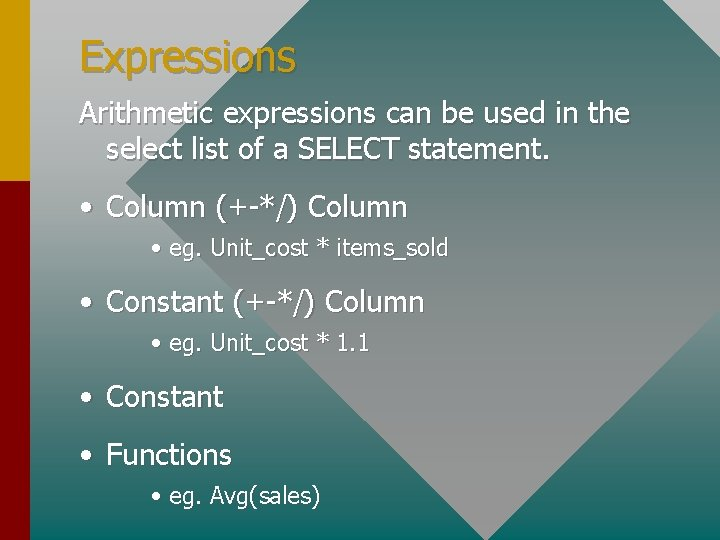Expressions Arithmetic expressions can be used in the select list of a SELECT statement. • Column (+-*/) Column • eg. Unit_cost * items_sold • Constant (+-*/) Column • eg. Unit_cost * 1. 1 • Constant • Functions • eg. Avg(sales)Expressions allow us. . . • To make projections • To do “What if” analyses • Alter reported data without altering the physical dataPrecedence Hierarchy of Arithmetic Operators Parentheses Multiplication, Division Addition, Subtraction (2+2*3)/4 = 2The Where Clause • Arithmetic (+-*/) /Comparative (><=, !=) Operators WHERE cost * 2 >= 20 WHERE state != ‘CA’ • • • Logical Operators (AND, OR, NOT) Ranges (BETWEEN, NOT BETWEEN) Lists (IN, NOT IN) WHERE state NOT IN (‘CA’, ‘MD’, ‘NJ’) • • Unknowns (IS NULL, IS NOT NULL) Character Matches (LIKE, NOT LIKE) WHERE au_lname LIKE (‘Gr%’) OR au_lname LIKE (‘_reen’)Precedence Hierarchy of Logical Operators Parentheses Multiplication, Division Addition, Subtraction NOT AND OR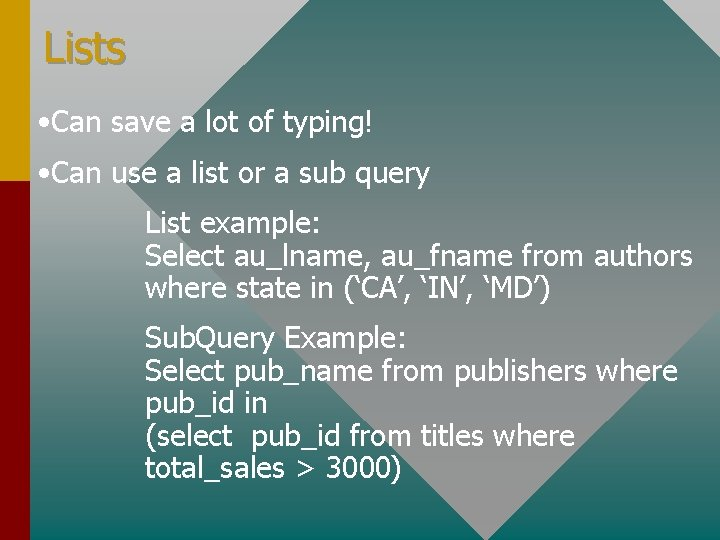Lists • Can save a lot of typing! • Can use a list or a sub query List example: Select au_lname, au_fname from authors where state in (‘CA’, ‘IN’, ‘MD’) Sub. Query Example: Select pub_name from publishers where pub_id in (select pub_id from titles where total_sales > 3000)Character Matches • % - 0 or more character • _ - 1 character • @ - lets you escape a wildcard • You can use 1 or more wildcards in a string. Eg. I%a = Indiana, Iowa, Ina-goda-da-vida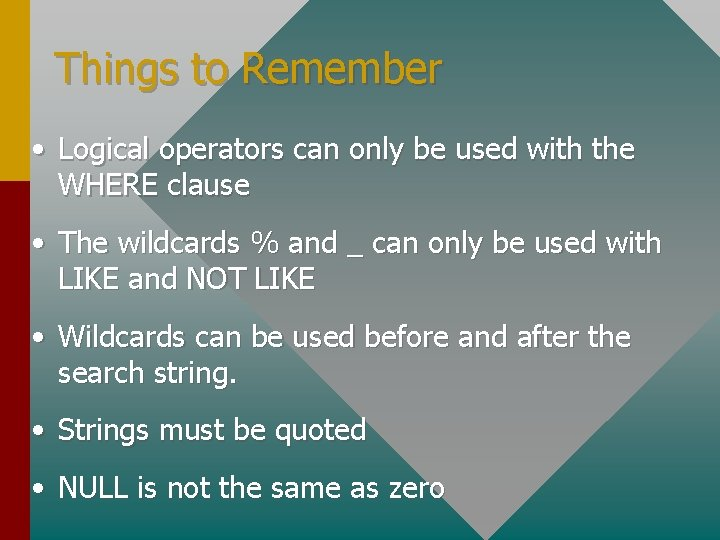Things to Remember • Logical operators can only be used with the WHERE clause • The wildcards % and _ can only be used with LIKE and NOT LIKE • Wildcards can be used before and after the search string. • Strings must be quoted • NULL is not the same as zero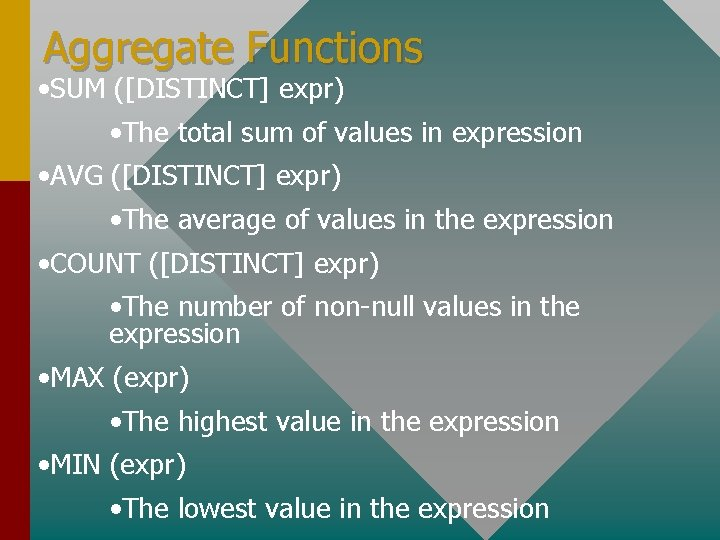Aggregate Functions • SUM ([DISTINCT] expr) • The total sum of values in expression • AVG ([DISTINCT] expr) • The average of values in the expression • COUNT ([DISTINCT] expr) • The number of non-null values in the expression • MAX (expr) • The highest value in the expression • MIN (expr) • The lowest value in the expressionSyntax SELECT avg(price * 2) from titles • returns the average of all prices * 2 SELECT sum (price) from titles • return the sum of the prices